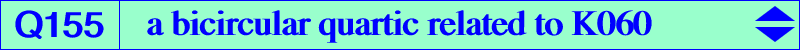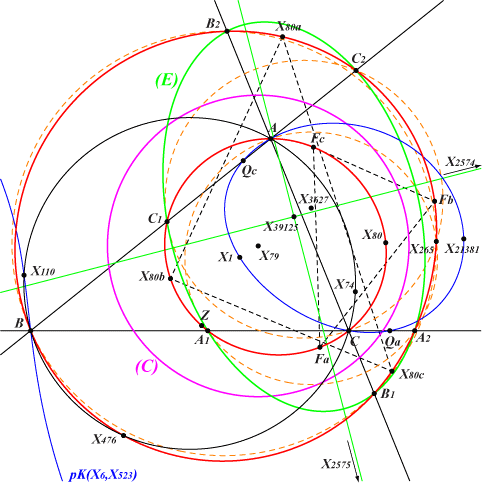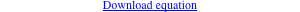too complicated to be written here. Click on the link to download a text file.X(80), X(265), X(476), X(39136), X(39139), X(39140) Z = X(39136) = QF-image of X(21381) extraversions of X(80) = QF-images of the excenters of ABC Fa, Fb, Fc = QF-images of Qa, Qb, Qc (cevians of X523) other points belowLet P be a point and Pa, Pb, Pc its reflections in the sidelines BC, CA, AB of ABC respectively. The lines APa, BPb, CPc concur (at Q) if and only if P and its isogonal conjugate P* lie on the Neuberg cubic K001 (see locus property 1). The locus of Q is K060 and P, P*, Q are collinear. Note that P* is the circum-center of triangle PaPbPc. The circles with diameters APa, BPb, CPc concur (at X) if and only if P and P* lie on K1155 = pK(X6, X523). (Angel Montesdeoca, see here in Spanish). X is in fact the QF-image of P as in Table 74 and, since pK(X6, X523) passes through the imaginary foci M1 and M2 of the MacBeath inconic, the locus of X must be a bicircular quartic, namely Q155. A remarkable common property : K060 and Q155 are both globally invariant under the inversion with pole X(79) which swaps X(80) and X(265). The circle of inversion is labelled (C) on the figure. Other properties of Q155 Q155 meets the sideline BC again at A1, A2 which lie on the circle passing through A and X(265), whose center also lies on the perpendicular at X(3627) to BC. The points B1, B2 on CA and C1, C2 on AB are defined likewise. These six points lie on a conic (E) passing through the infinite points of the circum-conic with perspector X(32). (E) is an ellipse when ABC is acutangle. The axes of (E) are parallel at its center X(39125) to the asymptotes of the Jerabek hyperbola. Two points on pK(X6, X523) collinear with X(1) have their QF-images collinear with X(79) and inverses about (C). This is the case of X(110), X(21381) on the cubic and their images X(476), X(39136) on the quartic.